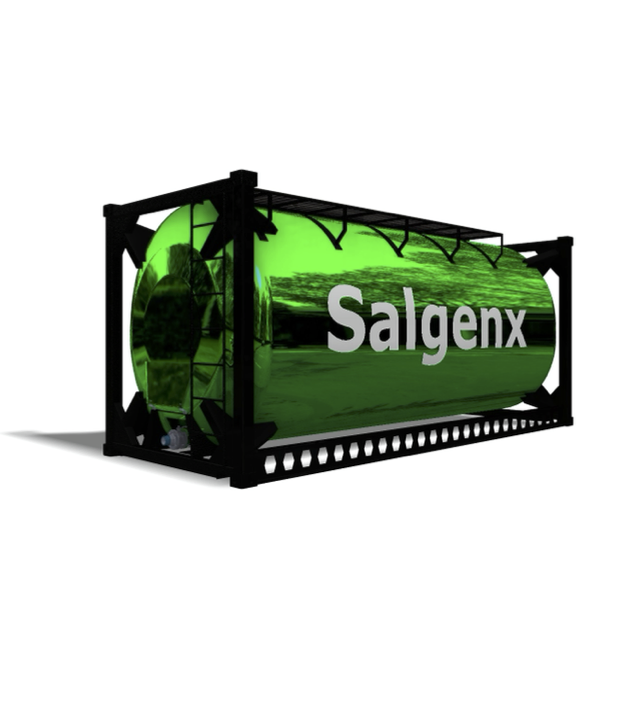# Electrochemistry Chapter 14 to 16

PDF Publication Title:

## Electrochemistry Chapter 14 to 16 ( electrochemistry-chapter-14-16 )

Text from PDF Page: 001

Ch.14-16 Electrochemistry Redox Reaction - the basics ox1 + red2 <=> red1 + ox2 Oxidizing Reducing Agent Agent Redox reactions: involve transfer of electrons from one species to another. Oxidizing agent (oxidant): takes electrons Reducing agent (reductant): gives electrons Redox Reaction - the basics Reduced Oxidized ox1 + red2 <=> red1 + ox2 Oxidizing Reducing Agent Agent Redox reactions: involve transfer of electrons from one species to another. Oxidizing agent (oxidant): takes electrons Reducing agent (reductant): gives electrons Fe3+ +V2+→Fe2++V3+ Balance Redox Reactions (Half Reactions) 1. Write down the (two half) reactions. 2. Balance the (half) reactions (Mass and Charge): a. Start with elements other than H and O. b. Balance O by adding water. c. balance H by adding H+. d. Balancing charge by adding electrons. (3. Multiply each half reaction to make the number of electrons equal. 4. Add the reactions and simplify.) Example: Balance the two half reactions and redox reaction equation of the titration of an acidic solution of Na2C2O4 (sodium oxalate, colorless) with KMnO4 (deep purple). MnO4-(aq) + C2O42-(aq) → Mn2+ (aq) + CO2(g) 16H+(aq) + 2MnO4-(aq) + 5C2O42-(aq) → 2Mn2+(aq) + 8H2O(l) + 10CO2(g) Example: Balance Sn2+ + Fe3+ <=> Sn4+ + Fe2+ Fe2+ + MnO4- <=> Fe3+ + Mn2+ Important Redox Titrants and the Reactions Oxidizing Reagents (Oxidants) (1) Potassium Permanganate MnO − +8H+ +5e− →Mn2+ +4H O 42 MnO − + 4H + + 3e− → MnO (s) + 2H O 422 MnO− +e− →MnO2− 44 1

PDF Image | Electrochemistry Chapter 14 to 16PDF Search Title:

Electrochemistry Chapter 14 to 16

Original File Name Searched:

lecture9-10.pdf

DIY PDF Search: Google It | Yahoo | Bing

Salgenx Redox Flow Battery Technology: Salt water flow battery technology with low cost and great energy density that can be used for power storage and thermal storage. Let us de-risk your production using our license. Our aqueous flow battery is less cost than Tesla Megapack and available faster. Redox flow battery. No membrane needed like with Vanadium, or Bromine. Salgenx flow battery

 CONTACT TEL: 608-238-6001 Email: greg@salgenx.com | RSS | AMP